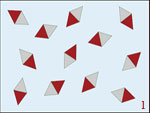Automatic translation Category: matter and particles
Updated November 11, 2015### Image: representation of the molecular wave function showing the border of the atoms in a molecule. Which begins and ends an atom? The atom is a field and it is the field lines which define its volume. No one has seen the fields of quantum physics, but it could look like this computer-generated image. When atoms bind them, their fields are deformed, this deformation is that characterizes bonds. The particles of the quantum theory are not "balls", but undulations, fields which have a wavelength, this wavelength is the size of the particle, and the field energy of the particle. credit image: T.A. Keith.### Video: The nucleon field. No optical device allows us to see the bustle of small particles inside a proton or a neutron, but the image layout, same false, is fundamental to understanding the concepts. So in this video, a simulation of the mathematical concept of the nucleon was conducted to allow us to make an intuition of what happens inside protons and neutrons. Credit: 1996 - Jean-François Colonna (Centre de Mathématiques appliquées de l'Ecole Polytechnique et France Télécom).

The wave function is one of the fundamental concepts of quantum mechanics.
It corresponds to the representation of the quantum state of a system in an infinite-dimensional basis.
The wave function gives every particle, typical interference properties of a wave.
In classical mechanics we represent the movement by particles which move in space, in quantum mechanics we represent the real and imaginary particles by wavefunctions.
These wave functions corresponding to stationary or non-stationary states (time-dependent) of energy.
In the standard model of particle physics, a hadron is composed of quarks and/or anti-quarks and gluons.
Subatomic particles constituting a hadron are called partons.
Quarks or antiquarks present in the hadron are called valence quarks while the quark-antiquark pairs and gluons that appear and disappear permanently in the hadron, are called virtual particles.
Gluons are the vectors of the strong force that holds quarks together.
Bosons are subatomic particles that transmit information different forces or interactions. Bosons are social particles, they like to mix, like light which mixes with the light, the photons are bosons.
The photon is the particle mediator the electromagnetic interaction.
The gluon is the messenger of the strong nuclear force, it confines quarks binding together very strongly.
Bosons Z 0 and W ± are the gauge bosons of the weak interaction.
The two categories of the nature of the particles are fermions and bosons.
In particle physics, the model of partons was proposed by Richard Feynman in 1969 to describe the structure of hadrons (protons, neutrons) and model the interactions with hadrons of high energy.
Partons are quarks, antiquarks and gluons that make up hadrons.
Quarks present in the hadron throughout its existence are called valence quarks, the opposite of virtual particles (quark-antiquark pairs and gluons) that appear and disappear permanently in the hadron. Gluons are the vectors of the strong force that holds quarks together.
A hadron is a compound of partons, subatomic particles governed by the strong interaction.
Fermions are subatomic particles (electrons, neutrinos and quarks) of the matter.
All matter that makes up the objects around us are made of fermions. Fermions are asocial particles, in other words they refuse to reduce their living space, this is why the matter is not compressible and that we can walk on the floor.
The two categories of nature particles are fermions and bosons.
The Hilbert space, David Hilbert (1862 - 1943), is a vector space equipped with a scalar product for measuring lengths and angles.
Hilbert space generalizes the notion of classical Euclidean space (two-dimensional plane and three-dimensional space) to spaces of any dimension, finite or infinite.
The Hilbert space is an abstract mathematical concept which allows to apply the techniques of mathematical analysis to all spaces. These techniques are used in theories of equations partial derivative, in quantum mechanics, Fourier analysis, thermodynamics.
 1997 © Astronoo.com − Astronomy, Astrophysics, Evolution and Earth science. Contact    Mentions légalesScale of the nanoparticles...Neutrino
and beta emission...Magnetic order
and magnetization...